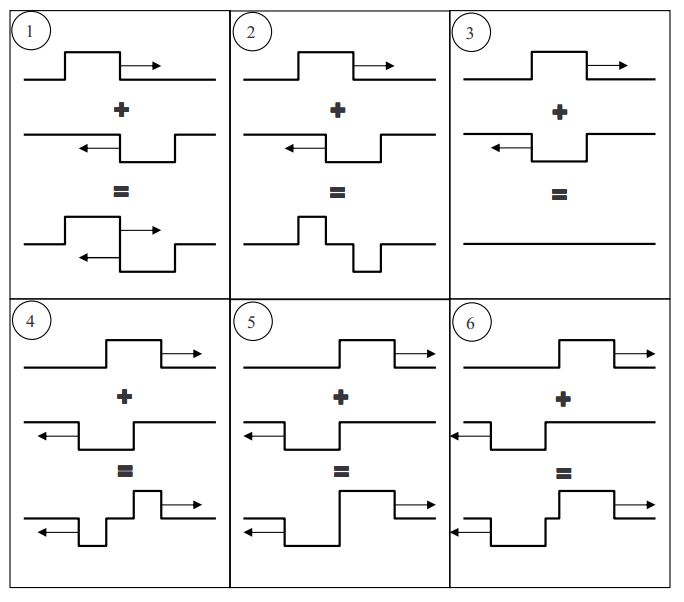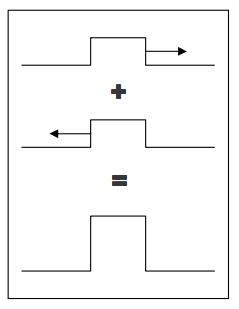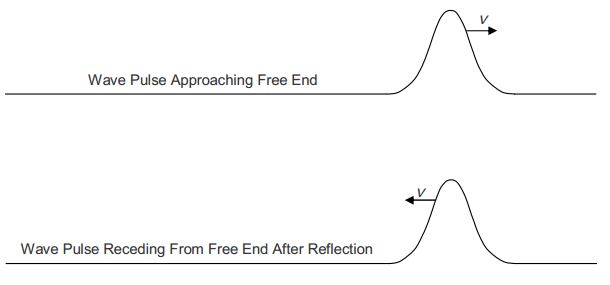$$\require{cancel}$$

# 30A: Wave Function, Interference, Standing Waves

In that two of our five senses (sight and sound) depend on our ability to sense and interpret waves, and in that waves are ubiquitous, waves are of immense importance to human beings. Waves in physical media conform to a wave equation that can be derived from Newton’s Second Law of motion. The wave equation reads:

$\frac{\partial ^2 y}{\partial x^2}=\frac{1}{v^2}\frac{\partial^2 y}{\partial t^2}\label{30-1}$

where:

$$y$$ is the displacement of a point on the medium from its equilibrium position,
$$x$$ is the position along the length of the medium,
$$t$$ is time, and
$$v$$ is the wave velocity.

Take a good look at this important equation. Because it involves derivatives, the wave equation is a differential equation. The wave equation says that the second derivative of the displacement with respect to position (treating the time $$t$$ as a constant) is directly proportional to the second derivative of the displacement with respect to time (treating the position $$x$$ as a constant). When you see an equation for which this is the case, you should recognize it as the wave equation.

In general, when the analysis of a continuous medium, e.g. the application of Newton’s second law to the elements making up that medium, leads to an equation of the form

$\frac{\partial^2 y}{\partial x^2}=\Big | \mbox{constant} \Big | \frac{\partial^2 y}{\partial x^2}$,

the constant will be an algebraic combination of physical quantities representing properties of the medium. That combination can be related to the wave velocity by

$\Big| \mbox{constant} \Big|=\frac{1}{v^2}$

For instance, application of Newton’s Second Law to the case of a string results in a wave equation in which the constant of proportionality depends on the linear mass density $$\mu$$ and the string tension $$F_T$$:

$\frac{\partial^2 y}{\partial x^2}=\frac{\mu}{F_T} \frac{\partial ^2 y}{\partial t^2}$

Recognizing that the constant of proportionality $$\frac{\mu}{F_T}$$ has to be equal to the reciprocal of the square of the wave velocity, we have

$\frac{\mu}{F_T}=\frac{1}{v^2}$

$v=\sqrt{\frac{F_T}{\mu}}\label{30-2}$

relating the wave velocity to the properties of the string. The solution of the wave equation $$\frac{\partial ^2 y}{\partial x^2}=\frac{1}{v^2} \frac{\partial ^2 y}{\partial t^2}$$ can be expressed as

$y \, =\space y_{\max} \cos (\frac{2\pi}{\lambda}x\pm \frac{2\pi}{\lambda} t+\phi)\label{30-3}$

where:

$$y$$ is the displacement of a point in the medium from its equilibrium position,
$$y_{\max}$$ is the amplitude of the wave,
$$x$$ is the position along the length of the medium,
$$\lambda$$ is the wavelength,
$$t$$ is time,
$$T$$ is the period, and
$$\phi$$ is a constant having units of radians. $$\phi$$ is called the phase constant.

A “$$-$$“ in the location of the “$$\pm$$” is used in the case of a wave traveling in the $$+x$$ direction and a “$$+$$” for one traveling in the $$–x$$ direction. Equation $$\ref{30-3}$$, the solution to the wave equation $$\frac{\partial ^2 y}{\partial x^2}=\frac{1}{v^2} \frac{\partial ^2 y}{\partial t^2}$$, is known as the wave function. Substitute the wave function into the wave equation and verify that you arrive at

$v=\frac{\lambda}{T} ,$

a necessary condition for the wave function to actually solve the wave equation. $$v=\frac{\lambda}{T}$$ is the statement that the wave speed is equal to the ratio of the wavelength to the period, a relation that we derived in a conceptual fashion in the last chapter.

At position $$x=0$$ in the medium, at time $$t=0$$, the wave function, equation $$\ref{30-3}$$, evaluates to

$y=y_{\max} \cos(\phi) \notag.\label{30-4}$

The phase of the cosine boils down to the phase constant $$\phi$$ whose value thus determines the value of $$y$$ at $$x=0$$, $$t=0$$. (Note that the “phase” of the cosine is the argument of the cosine—that which you are taking the cosine of.) The value of the phase constant $$\phi$$ is of no relevance to our present discussion so we arbitrarily set $$\phi = 0$$. Also, on your formula sheet, we write the wave function only for the case of a wave traveling in the $$+x$$ direction, that is, we replace the “$$\pm$$” with a “$$-$$” . The wave function becomes

$y=y_{\max} \cos \Big (\frac{2\pi}{\lambda}x-\frac{2\pi}{T} t)\label{30-5}$

This is the way it appears on your formula sheet. You are supposed to know that this corresponds to a wave traveling in the +x direction and that the expression for a wave traveling in the $$–x$$ direction can be arrived at by replacing the “$$-$$ “ with a “$$+$$”.

# Interference

Consider a case in which two waveforms arrive at the same point in a medium at the same time. We’ll use idealized waveforms in a string to make our points here. In the case of a string, the only way two waveforms can arrive at the same point in the medium at the same time is for the waveforms to be traveling in opposite directions:The two waveforms depicted in the diagram above are “scheduled” to arrive at point $$A$$ at the same time. At that time, based on the waveform on the left alone, point $$A$$ would have a displacement $$+h$$, and based on the waveform on the right alone, point $$A$$ would have the displacement $$–h$$. So, what is the actual displacement of point $$A$$ when both waveforms are at point $$A$$ at the same time? To answer that, you simply add the would-be single-waveform displacements together algebraically (taking the sign into account). One does this point for point over the entire length of the string for any given instant in time. In the following series of diagrams we show the point-for-point addition of displacements for several instants in time.The phenomenon in which waves traveling in different directions simultaneously arrive at one and the same point in the wave medium is referred to as interference. When the waveforms add together to yield a bigger waveform,the interference is referred to as constructive interference. When the two waveforms tend to cancel each other out,the interference is referred to as destructive interference.

# Reflection of a Wave from the End of a Medium

Upon reflection from the fixed end of a string, the displacement of the points on a traveling waveform is reversed.The fixed end, by definition, never undergoes any displacement.

Now we consider a free end. A fixed end is a natural feature of a taut string. A free end, on the other hand, an idealization, is at best an approximation in the case of a taut string. We approximate a free end in a physical string by means of a drastic and abrupt change in linear density. Consider a rope, one of which is attached to the wave source, and the other end of which is attached to one end of a piece of thin, but strong, fishing line. Assume that the fishing line extends through some large distance to a fixed point so that the whole system of rope plus fishing line is taut. A wave traveling along the rope, upon encountering the end of the rope attached to the thin fishing line, behaves approximately as if it has encountered a free end of a taut rope.In the case of sound waves in a pipe, a free end can be approximated by an open end of the pipe.

Enough said about how one might set up a physical free end of a wave medium, what happens when a wave pulse encounters a free end? The answer is, as in the case of the fixed end, the waveform is reflected, but this time, there is no reversal of displacements.# Standing Waves

Consider a piece of string, fixed at both ends, into which waves have been introduced. The configuration is rich with interference. A wave traveling toward one end of the string reflects off the fixed end and interferes with the waves that were trailing it. Then it reflects off the other end and interferes with them again. This is true for every wave and it repeats itself continuously. For any given length, linear density, and tension of the string, there are certain frequencies for which, at, at least one point on the string, the interference is always constructive. When this is the case, the oscillations at that point (or those points) on the string are maximal and the string is said to have standing waves in it. Again, standing waves result from the interference of the reflected waves with the transmitted waves and with each other. A point on the string at which the interference is always constructive is called an antinode. Any string in which standing waves exist also has at least one point at which the interference is always destructive. Such a point on the string does not move from its equilibrium position. Such a point on the string is called a node.

It might seem that it would be a daunting task to determine the frequencies that result in standing waves. Suppose you want to investigate whether a point on a string could be an antinode. Consider an instant in time when a wave crest is at that position. You need to find the conditions that would make it so that in the time it takes for the crest to travel to one fixed end of the string, reflect back as a trough and arrive back at the location in question; a trough, e.g. one that was trailing the original crest, propagates just the right distance so that it arrives at the location in question at the same time. As illustrated in the next chapter, the analysis that yields the frequencies of standing waves is easier than these timing considerations would suggest.